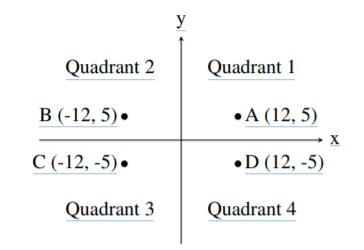TORONTO KIDS COMPUTER CLUB | Thursday 18:30 Python Practice 22.05.19.
20658

# Thursday 18:30 Python Practice 22.05.19.

## 23 May Thursday 18:30 Python Practice 22.05.19.

Question 1: （difficulty Level: *)

A common problem in mathematics is to determine which quadrant a given point lies in. There are four quadrants, numbered from 1 to 4, as shown in the diagram below:For example, the point A, which is at coordinates (12, 5) lies in quadrant 1 since both its x and y values are positive, and point B lies in quadrant 2 since its x value is negative and its y value is positive.

Your job is to take a point and determine the quadrant it is in. You can assume that neither of the two coordinates will be 0.

Input Specification
The first line of input contains the integer x (−1000 ≤ x ≤ 1000; x 6= 0). The second line of input contains the integer y (−1000 ≤ y ≤ 1000; y 6= 0).

Output Specification
Output the quadrant number (1, 2, 3 or 4) for the point (x, y).

Sample Input 1
12
5

Output for Sample Input 1
1

Sample Input 2
9
-13

Output for Sample Input 2
4

Question 2:（difficulty Level: **)

You have been asked to take a small icon that appears on the screen of a smart telephone and scale it up so it looks bigger on a regular computer screen.

The icon will be encoded as characters (x and *) in a 3 × 3 grid as follows:

```*x*
xx
* *```

Write a program that accepts a positive integer scaling factor and outputs the scaled icon. A scaling factor of k means that each character is replaced by a k × k grid consisting only of that character.

Input Specification
The input will be a positive integer k such that k < 25.

Output Specification
The output will be 3k lines, which represent each individual line scaled by a factor of k and repeated k times. A line is scaled by a factor of k by  replacing each character in the line with k copies of the character.

Sample Input
3

Output for Sample Input

```***xxx***
***xxx***
***xxx***
xxxxxx
xxxxxx
xxxxxx
***   ***
***   ***
***   ***```